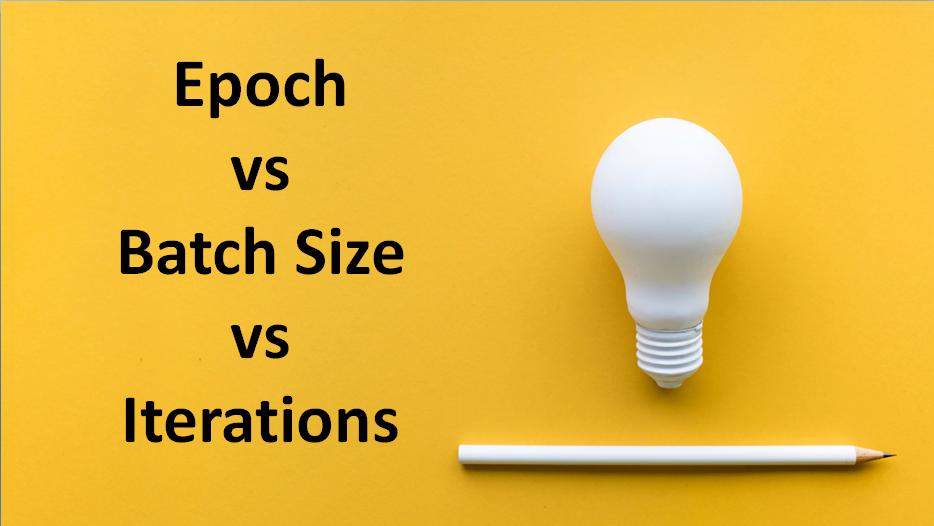/  Technology   /  Epoch vs Batch Size vs Iterations## Epoch vs Batch Size vs Iterations

Epoch, Batch size and iterations they all look similar right. You will come to know of them in a short while.

Before that let’s know few terms.

#### Samples

Sample consists of inputs that are used into algorithm and outputs used to compare the prediction and calculate an error. A sample is single row of data.

We can form a dataset by using samples.

Gradient means the rate of inclination or declination of a slope.

Descent means the instance of descending.

It is an iterative optimization algorithm used in machine learning to find the best results (minima of a curve).

It is an iterative algorithm because it gives multiple results to get the optimal results.

In most cases, it is not possible to input all the training data to an algorithm in one pass.

An epoch is when an entire dataset is passed forward and backward through the neural network exactly once. If the entire dataset cannot be passed into the algorithm at once, it must be divided into several small Batches. Batch size is the total number of training samples present in a single batch. An iteration is a single gradient update during training. The number of iterations is the number of batches needed to complete one epoch.

So, if a dataset includes 2,000 images split into mini-batches of 500 images, it will take 4 iterations to complete a single epoch.

#### What is the right number of epochs?

During each pass through the network, the weights are updated and the curve goes from underfitting, to optimal, to overfitting. There is no magic rule for choosing the number of epochs — this is a hyperparameter that must be determined before training begins.

#### What is the right batch size?

Batch size is also a hyperparameter with no magic rule of thumb. Choosing a batch size that is too small will introduce a high degree of variance within each batch as it is unlikely that a small sample is a good representation of the entire dataset. On the other hand, if a batch size is too large, it may not fit in memory of the compute instance used for training and it will have the tendency to overfit the data. It’s important to note that batch size is affected by other hyperparameters such as learning rate so the combination of these hyperparameters is as important as batch size itself.

A commonly observed batch size is to use the square root of the size of the dataset.